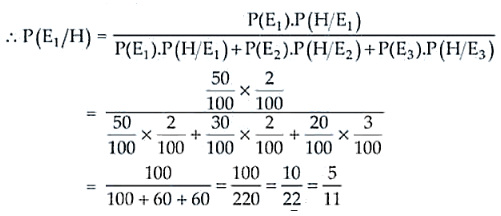# An item is manufactured by three machines A, B and C.

Question:

An item is manufactured by three machines A, B and C. Out of the total number of items manufactured during a specified period, 50% are manufactured on A,

30% on B and 20% on C. 2% of the items produced on A and 2% of items produced on B are defective, and 3% of these produced on C are defective. All the items

are stored at one godown. One item is drawn at random and is found to be defective. What is the probability that it was manufactured on machine A?

Solution:

Let’s consider:

E1 = The event that the item is manufactured on machine A

E2 = The event that the item is manufactured on machine B

E3 = The event that the item is manufactured on machine C

Let H be the event that the selected item is defective.

Thus, by using Baye’s theorem we have

P(E1) = 50/100, P(E2) = 30/100, P(E3) = 20/100

P(H/E1) = 2/100, P(H/E2) = 2/100 and P(H/E3) = 3/100Therefore, the required probability is 5/11.